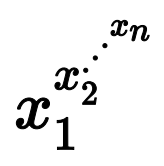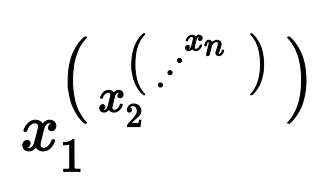시간 제한 메모리 제한 제출 정답 맞은 사람 정답 비율
2 초 512 MB 25 10 6 50.000%

## 문제

Suppose that we have a non empty list of positive integers: [x1, x2, ..., xN]. The power tower corresponding to this list is the number defined as:, that isPower towers can be very big numbers, even when the list consists of only a few small integers. For example, the power tower of the list [2, 3, 2] is equal to 29 = 512, and that of [5, 2, 3, 2] is equal to 5512 and has 358 decimal digits. In this task, you will have to compute power towers modulo a given positive integer M.

## 입력

The first line of the input will contain two positive integers: T and M. Each of the following T lines will contain a positive integer N, followed by a list of N positive integers x1, x2, ... xN. There will be exactly one space separating consecutive numbers in all lines of the input.

1 ≤ T ≤ 1 000, the sum of the lengths N of all lists will not exceed 1 000 000, and 1 ≤ xi ≤ 1 000 000. 2 ≤ M ≤ 1 000 000.

## 출력

The output must contain T lines, each containing a single non-negative integer. The number in line k must be equal to the result of the power tower for the k-th given list modulo M.

## 예제 입력 1

9 10
1 42
2 2 2
4 2 2 2 2
5 2 2 2 2 2
3 3 4 5
3 4 3 6
3 7 6 21
3 937640 767456 981242
10 42 1 17 17 17 17 17 17 17 17


## 예제 출력 1

2
4
6
6
1
4
1
0
2


## 힌트

In this example, as M = 10, we are interested in the last decimal digit of each power tower. Notice that, except for the first three power towers and the last one, all the others are too large to be represented with 32 or 64 bit integers.